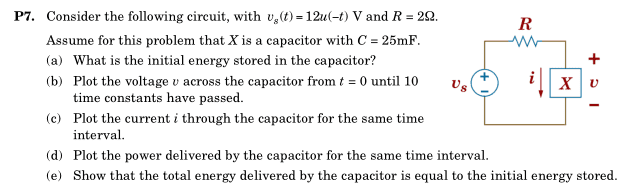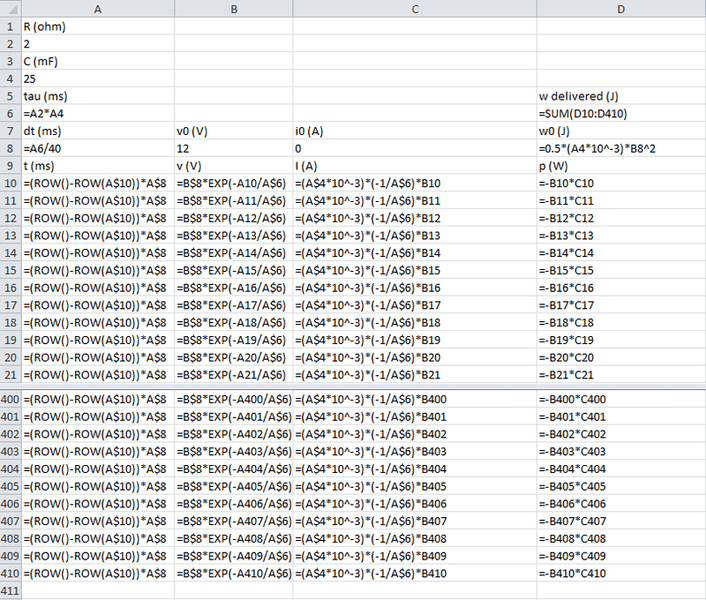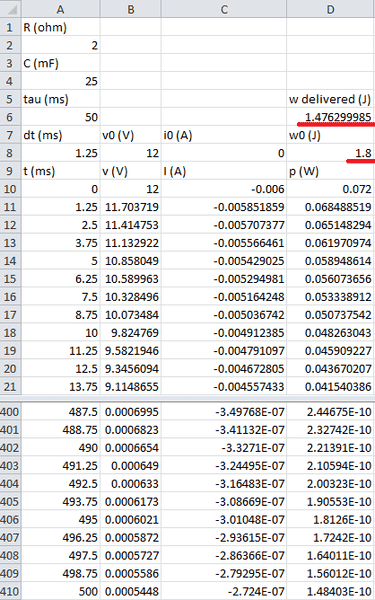# Solving RC circuit in excel

• Engineering

## Homework Statement## Homework Equations

v(t) = v0*e^(-t/RC)
i(t) = C*(dv/dt)
E = .5*C*V^2
P=v*i

## The Attempt at a Solution

I'm having trouble with part e. As far as I can tell, all of my equations are correct, but the total energy delivered is notably less than the initial energy; I was hoping someone could point out my mistake.
Here's what I've got:gneill
Mentor
I can see a few odd things. First, the initial current should be 6A. If you're trying to use i = C*dv/dt to calculate it you'll need a dV value, the change in voltage over the timestep, not the current value of V. And you'll need to use dt, not $\tau$. But then, why not write an explicit formula for the current as you did for the voltage? i(t) = Io exp(-t/$\tau$).

The sum of the instantaneous power over time is not the total power delivered. Each of those power points needs to be multiplied by the timestep size to determine the energy delivered in that timestep. So for the timesptep at time t, the energy delivered is approximately v(t)*i(t)*dt.

Thank you very much for the response. I seem to be getting the correct answer (~1.845 J which is within my allowed margin of error) now when each power value is multiplied by dt. However, I'm a bit confused as to why the initial current would be 6A, why is it not the steady state value of 0A?

gneill
Mentor
Thank you very much for the response. I seem to be getting the correct answer (~1.845 J which is within my allowed margin of error) now when each power value is multiplied by dt. However, I'm a bit confused as to why the initial current would be 6A, why is it not the steady state value of 0A?

If I understood the circuit properly, at time t=0 the voltage source switches from supplying 12V to supplying 0V, or in other words, it becomes a short circuit. This being so, the capacitor is left charged up with 12V on it, and that 12V "sees" a resistance of 2.0 Ohms. 12V/2Ω = 6.0 Amps. That's your initial current.

If I understood the circuit properly, at time t=0 the voltage source switches from supplying 12V to supplying 0V, or in other words, it becomes a short circuit. This being so, the capacitor is left charged up with 12V on it, and that 12V "sees" a resistance of 2.0 Ohms. 12V/2Ω = 6.0 Amps. That's your initial current.

Thanks, that makes sense. I forgot that the current through a capacitor can change instantaneously.